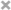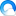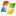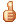# 使用JavaScript为网站显示运行时间代码案列，附代码！

Begin！！！！

## 方法一：

.1.只显示运行天数(☄⊙ω⊙)☄

```<!DOCTYPE html>
<html>

<meta charset="UTF-8">
<title>只显示运行天数</title>

<body>
<!--只显示运行天数-->
<!--其中11是月份，22是日期，2017是年数，根据自己情况修改一下即可，然后把代码复制到你想要显示的地方。-->
本站已安全运行了
<b style="color: red;"><strong>
<script language="JavaScript" type="text/javascript">
var urodz = new Date("11/22/2017");
var now = new Date();
var ile = now.getTime() - urodz.getTime();
var dni = Math.floor(ile / (1000 * 60 * 60 * 24));
document.write(+dni)
</script>
</strong></b>天

</body>

</html>```

## 方法二：

.2.显示XX年XX天XX时XX秒(☄⊙ω⊙)☄

```<!DOCTYPE html>
<html>

<meta charset="UTF-8">
<title>显示XX年XX天XX时XX秒，秒数显示为一秒一秒的走动</title>

<body>
<!--第二个的代码相对第一种的较多，不过显示靓丽，至于修改也是拷贝到自己想要显示的地方修改个日期即可。-->
阁主博客！<br /> 网站稳定运行：
<span id="htmer_time" style="color: crimson;"></span>

<!--JavaScript部分-->
<script>
function secondToDate(second) {
if(!second) {
return 0;
}
var time = new Array(0, 0, 0, 0, 0);
if(second >= 365 * 24 * 3600) {
time = parseInt(second / (365 * 24 * 3600));
second %= 365 * 24 * 3600;
}
if(second >= 24 * 3600) {
time = parseInt(second / (24 * 3600));
second %= 24 * 3600;
}
if(second >= 3600) {
time = parseInt(second / 3600);
second %= 3600;
}
if(second >= 60) {
time = parseInt(second / 60);
second %= 60;
}
if(second > 0) {
time = second;
}
return time;
}
</script>
<script type="text/javascript" language="javascript">
function setTime() {
var create_time = Math.round(new Date(Date.UTC(2017, 11, 22, 18, 20, 02)).getTime() / 1000);
var timestamp = Math.round((new Date().getTime() + 8 * 60 * 60 * 1000) / 1000);
currentTime = secondToDate((timestamp - create_time));
currentTimeHtml = currentTime + '年' + currentTime + '天' +
currentTime + '时' + currentTime + '分' + currentTime +
'秒';
document.getElementById("htmer_time").innerHTML = currentTimeHtml;
}
setInterval(setTime, 1000);
</script>
<!--有关CSS样式自行修改，上面代码中2017, 11, 22, 18, 20, 02分别对应是 年、月、日、时、分、秒，根据自己需要的值修改即可。自此本文讲述完成，如有问题可在下方留言。。

The End-->
</body>

</html>```

## 预览效果：### 评论列表

1.屋檐【评论达人 LV.6】  @回复

很棒的资料、老衲收藏。择日再加到自己站点上！